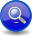#Boost C++ Libraries

...one of the most highly regarded and expertly designed C++ library projects in the world.

##### Epsilon (`eps`)
###### Description

The Epsilon (`eps`) is a multi-purpose parser that returns a zero length match.

###### Simple Form

In its simplest form, `eps` matches the null string and always returns a match of zero length:

```eps // always returns a zero-length match
```

This form is usually used to trigger a semantic action unconditionally. For example, it is useful in triggering error messages when a set of alternatives fail:

```r = a | b | c | eps[error()]; // Call error if a, b, and c fail to match
```
###### Semantic Predicate

Semantic predicates allow you to attach a conditional function anywhere in the grammar. In this role, the epsilon takes a Lazy Argument that returns `true` or `false`. The Lazy Argument is typically a test that is called to resolve ambiguity in the grammar. A parse failure will be reported when the Lazy Argument result evaluates to `false`. Otherwise an empty match will be reported. The general form is:

```eps(f) >> rest;
```

The Lazy Argument `f` is called to do a semantic test (say, checking if a symbol is in the symbol table). If test returns true, `rest` will be evaluated. Otherwise, the production will return early with a no-match without ever touching rest.

```// forwards to <boost/spirit/home/qi/auxiliary/eps.hpp>
#include <boost/spirit/include/qi_eps.hpp>
```

Also, see Include Structure.

###### Namespace

Name

```boost::spirit::eps // alias: boost::spirit::qi::eps```

###### Model of

Notation

`f`

A Lazy Argument that evaluates `bool`.

###### Expression Semantics

Semantics of an expression is defined only where it differs from, or is not defined in `PrimitiveParser`.

Expression

Semantics

`eps`

Match an empty string (always matches).

`eps(f)`

If `f` evaluates to `true`, return a zero length match.

Expression

Attribute

`eps`

`unused`

###### Complexity

For plain (`eps`) the complexity is O(1). For Semantic predicates (`eps(f)`) the complexity is defined by the function `f`.

###### Example
NoteThe test harness for the example(s) below is presented in the Basics Examples section.

Some using declarations:

```using boost::spirit::qi::eps;
using boost::spirit::qi::int_;
using boost::spirit::qi::_1;
namespace phx = boost::phoenix;
```

Basic `eps`:

```test_parser("", eps); // always matches
```

This example simulates the "classic" `if_p` parser. Here, `int_` will be tried only if the condition, `c`, is true.

```bool c = true; // a flag
test_parser("1234", eps(phx::ref(c) == true) >> int_);
```

This example simulates the "classic" `while_p` parser. Here, the kleene loop will exit once the condition, c, becomes true. Notice that the condition, ```c, is turned to ```false``` when we get to parse ```4`.

```test_phrase_parser("1 2 3 4",
*(eps(phx::ref(c) == true) >> int_[phx::ref(c) = (_1 == 4)]));
```